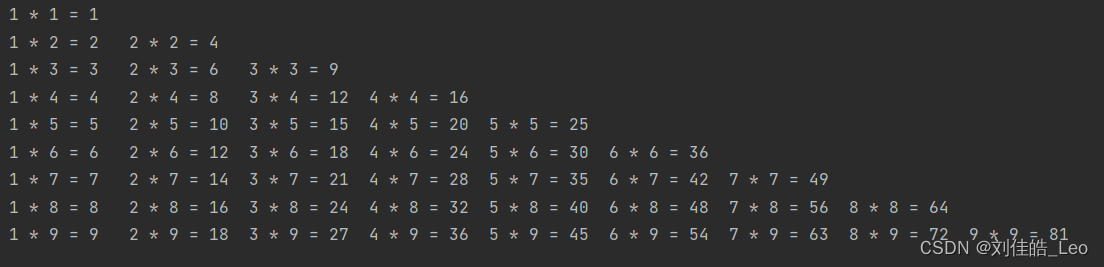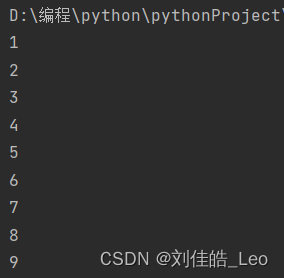# 【Python】9*9乘法口诀表（while、for两种循环）

14天阅读挑战赛

1.第一个是while循环。

2.代码不会局限于一种思路，第二种方法用到了用for循环。

### 1.第一个是while循环。

``````#设置j初始值
j = 1
while j <= 9:
#设置i初始值
i = 1
#让i<=9
while i <= j:
print(f'{i} * {j} = {i*j}', end='\t')
#i = i + 1的简写
i += 1
# 这个print的作用是换行
print()
j += 1````````````j = 1
while j <= 9:
print(j)
j += 1``````end='\t'，它的作用是取消换行。下面的代码是内循环的简写，跟外循环一样。

``````i = 1
while i <= 9:
print(i, end='\t')
i += 1``````

### 2.代码不会局限于一种思路，第二种方法用到了用for循环，

format()用于格式化方法，即用来控制字符串和变量的显示效果。

format()的使用方法

<模板字符串>.format(<逗号分隔的参数>)

``````#range函数的取值范围是（初始值，结束值-1）
for i in range(1, 10):
for j in range(1, i+1):
print('{}x{}={}\t'.format(j, i, i*j), end='')
#作用也是换行
print()``````

(1条消息) 用python做个九九乘法表-够简单,能学会_曾亲桂林的博客-CSDN博客